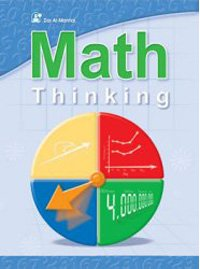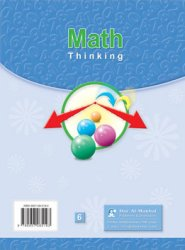+962 (6) 5533889
info@manhal.com
+962 (78) 7000522
support@manhal.com

# Math Thinking Level 06 book

• Book
• Description
• Related
• Rating
(0)
••8663
0
0
0
• Math Thinking 6 is one of Dar Al Manhal educational books that comes within Math Thinking curriculum which includes 6 that teach children mathematics basics in English language.

The book, Math Thinking 6 is divided into twelve units which include billions, and the calculations of adding and subtracting within.

The curriculum, Math Thinking 6, also teaches multiplication and division by (10,100,1000), decimal fractions (adding, subtracting, multiplication and division), properties of mathematical calculations, number theory, fractions, algebra, measurement, calculating the circumference, area, surface and volume. It also presents teaches ratios, percentages, integers, and probability and statistics.

The book, Math Thinking 6, also includes solving mathematical problems of each unit to test what children have learned from it.

160
Soft Cover
Product Dimensions and Weight
21 cm
28 cm
465 Gm
2006/5/1286
6
One
\$ 8.8
• Math
• Ammar Dkeidek And Others
• 2006
• English
• 9-11 Year
x
\$220